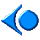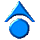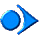We mentioned in a previous example that, after the the given array segment is partitioned into two subarrays, two threads are created to sort the subarrays, one for each subarray. More precisely, when a thread receives an array segment, this thread partition the segment into two, and creates two child threads to sort the left and right subarrays. Then, the parent simply waits until both of its child threads complete.

Based on the observation above, it is clear that a thread must receive the left and right bounds of the array segment. The array itself can be a global object; however, we prefer to pass it as an argument. Therefore, we may define the QuickSortThread class as follows, and save this interface into file quicksort.h

 ```#include "ThreadClass.h" class QuickSortThread : public Thread { public: // constructor QuickSortThread(int Lowerbound, int Upperbound, int Array[]); private: void ThreadFunc(); // thread body int lowerbound; // lower bound of the sub-array to be sorted int upperbound; // upper bound of the sub-array to be sorted int *a; // pointing to array to be sorted }; ``` Click here to download this file (quicksort.h)

Next, let us turn our attention to the implementation of this QuickSortThread class. In fact, what we have to do is to write a constructor and fill method ThreadFunc() with the statements that will be executed by this thread. The constructor is quite simple. The base class has a char variable ThreadName for us to name a thread. Thus, the constructor also gives a name to the thread. Note that the lower and upper bounds are part of the thread name. The sorting step can be found in any data structures or algorithms textbooks. Once the pivot point is found, two threads are created and run, one for each array section. Since we would prefer to separate the interface and the implementation, this implementation will be stored in file quicksort.cpp. As a result, at the beginning of this file, we need to include the interface file quicksort.h as shown below.

 ```#include #include "quicksort.h" QuickSortThread::QuickSortThread(int Lowerbound, int Upperbound, int Array[]) :lowerbound(Lowerbound), upperbound(Upperbound), a(Array) { ThreadName.seekp(0, ios::beg); ThreadName << "Sorting" << '(' << Lowerbound << ':' << Upperbound << ')' << '\0'; } void QuickSortThread::ThreadFunc() { Thread::ThreadFunc(); int pivot = a[upperbound]; // pick up pivot value int left = lowerbound - 1, // scan index from left side right = upperbound, // scan index from right side tmp, pivotIndex; QuickSortThread *leftSortThread, // recursive sorting threads *rightSortThread; if (lowerbound >= upperbound) Exit(); //return and recursion ends while (left < right) { // partition loop do { left++;} while (a[left] < pivot); do { right--;} while (a[right] > pivot); if (left < right ) { tmp = a[left]; a[left] = a[right]; a[right] = tmp; } } pivotIndex = left; // put the pivot back tmp = a[pivotIndex]; a[pivotIndex] = pivot; a[upperbound] = tmp; // start the "recursive threads" leftSortThread = new QuickSortThread(lowerbound, pivotIndex - 1, a); leftSortThread->Begin(); rightSortThread = new QuickSortThread(pivotIndex + 1, upperbound, a); rightSortThread->Begin(); leftSortThread->Join(); // wait for the child threads rightSortThread->Join(); Exit(); // done and exit } ``` Click here to download this file (quicksort.cpp)

 ```#include #include "quicksort.h" int main(int argc, char *argv[]) { QuickSortThread *quicksortthread; int *array; int arraySize, i; cout << "Please input array size:" << endl; cin >> arraySize; array = new int[arraySize]; // create the array cout << "Please input the array elements: " << endl; for (i = 0; i < arraySize; i++) cin >> array[i]; cout << "Before quicksort, the array is:" << endl; for (i = 0; i < arraySize; i++) cout << array[i] << " "; cout << endl; // start the quicksort thread quicksortthread = new QuickSortThread(0, arraySize - 1, array); quicksortthread->Begin(); // wait for the quicksort thread to finish quicksortthread->Join(); cout << "After quicksort, the array is:" << endl; for (i = 0; i < arraySize; i++) cout << array[i] << " "; cout << endl; Exit(); return 0; } ``` Click here to download this file (quicksort-main.cpp)